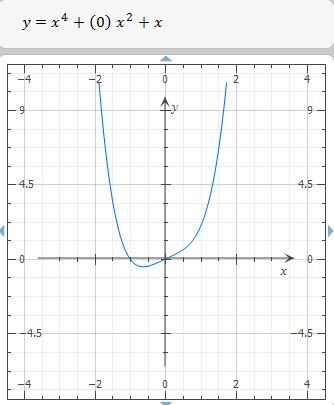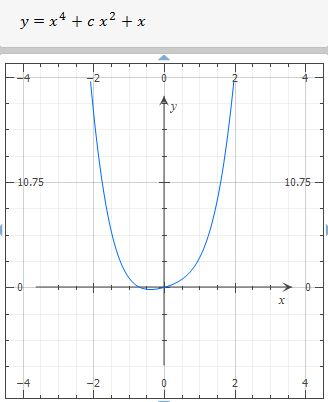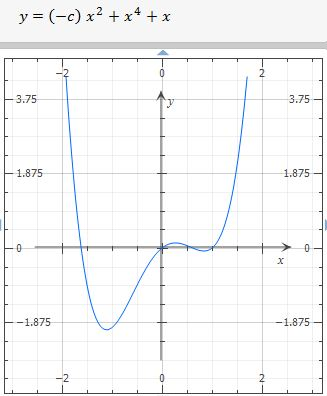# Investigate the family of curves given by the equation (x) = x^4 + cx^2 + x. Start by determining...

## Question:

Investigate the family of curves given by the equation {eq}\displaystyle f (x) = x^4 + cx^2 + x \\ {/eq}. Start by determining the transitional value of at which the number of inflection points changes. Then graph several members of the family to see what shapes are possible. There is another transitional value of c at which the number of critical numbers changes. Try to discover it graphically. Then prove what you have discovered.

## Inflection point

If a polynomial function with one independent variable has a constant, i.e. {eq}C {/eq}, then we have a family of the functions, because for each {eq}C {/eq} we have a specific or particular function, also, functions have minimum and maximum point at first derivative is zero, and inflection points where their second derivative is zero, remember, if the second derivative is positive the function is concave up and if the second derivative is negative, the function is concave down.

The function is:

{eq}\ f(x) = x^4 + cx^2 + x \\ {/eq}

Its first edrivative is:

{eq}\displaystyle \ f'(x)= 4\,{x}^{3}+2\,cx+1 \\ {/eq}

Its second derivative is:

{eq}\displaystyle \ f''(x) = 12\,{x}^{2}+2\,c \\ {/eq}

Inflection point at:

{eq}\displaystyle 0= \ f''(x) \; \Rightarrow \; 0= 12\,{x}^{2}+2\,c \\ \displaystyle 0=2(6x^2+c) \; \Rightarrow \; 0=6x^2+c \\ \displaystyle x^2=\frac{-c}{6} \; \Rightarrow \; x= \sqrt{\frac{-c}{6}} \text{ , } \; c \; \in \; (-\infty , 0 ) \; \; \; \; \text{&} \; \; \; \; x=- \sqrt{\frac{-c}{6}} \text{ , } \; c \; \in \; (-\infty , 0 ) \\ {/eq}

Conclusion: the inflection points depends on {eq}c {/eq} , if {eq}c {/eq} is positive, the function hasn't inflection point, and if {eq}c=0 {/eq} the function has only one inflection point {eq}\displaystyle 0=6x^2+c \; \Rightarrow \; 0=6x^2+0 \; \Rightarrow \; 6x^2=0 \; \Rightarrow \; x=0 \\ {/eq}

Graph